# MATH 251: Calculus 3, SET8

## 16: Vector Calculus

### 16.5: Curl and Divergence

These problems are done with the CAS. See Hand Solutions for details.

#### 1. [1109/2]

syms x y z real
F = [0 x^3 * y * z^2 y^4 * z^3]
F =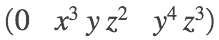curl_F = curl(F, [x y z])' % MATLAB's curl command returns a column vector, so we transpose.
curl_F =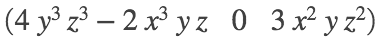div_F = divergence(F, [x y z])
div_F =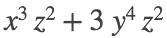#### 2. [1109/4]

syms x y z real
F = [sin(y*z) sin(x*z) sin(x*y)]
F =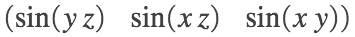curl_F = curl(F, [x y z])'
curl_F =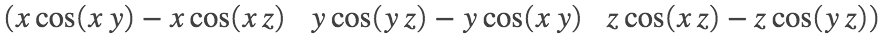div_F = divergence(F, [x y z])
div_F = 0

#### 3. [1109/6]

syms x y z real
F = [log(2*y + 3*z) log(x + 3*z) log(x + 2*y)]
F =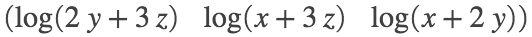curl_F = curl(F, [x y z])'
curl_F =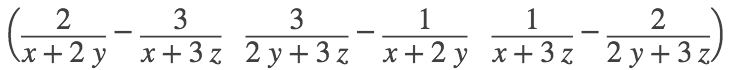div_F = divergence(F, [x y z])
div_F = 0

#### 4. [1109/8]

syms x y z real
F = [atan(x*y) atan(y*z) atan(x*z)]
F =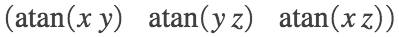curl_F = curl(F, [x y z])'
curl_F =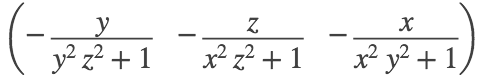div_F = divergence(F, [x y z])
div_F =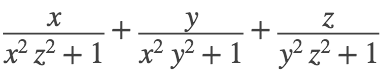#### 5. [1109/10]

syms x y z real
F = [x y 0] % This is the vector field depicted in the figure.
F =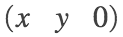curl_F = curl(F, [x y z])'
curl_F =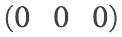div_F = divergence(F, [x y z])
div_F = 2
% (a) So div(F) = 2 > 0,
% (b) and curl(F) = [0 0 0], the zero vector.

#### 6. [1109/14]

syms x y z real
F = [x*y*z^4 x^2*z^4 4*x^2*y*z^3]
F =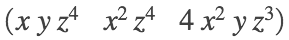curl_F = curl(F, [x y z])' % Since curl(F) is not the zero vector on R^3, F is not conservative.
curl_F =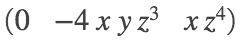f = potential(F, [x y z]) % Indeed, F has no potential function.
f = NaN

#### 7. [1109/16]

syms x y z real
F = [1 sin(z) y*cos(z)]
F =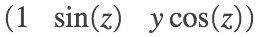curl_F = curl(F, [x y z])' % Since curl(F) is the zero vector on R^3, F is conservative.
curl_F =f = potential(F, [x y z]) % Here is a potential function f for vector field F.
f =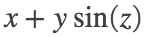#### 8. [1109/18]

syms x y z real
F = exp(x)*[sin(y*z) z*cos(y*z) y*cos(y*z)]
F =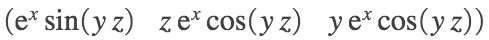curl_F = curl(F, [x y z])' % Since curl(F) is the zero vector on R^3, F is conservative.
curl_F =f = potential(F, [x y z]) % Here is a potential function f for vector field F.
f =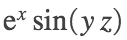#### 9. [1109/20]

Let F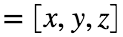. Assume there exists a vector field G on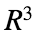such that curl(G) = F. Then by Theorem 11 on page 1106 of the textbook, div(curl(G)) = div(F) = 0. But div(F) = 3 (see below), a contradiction. Hence there is no such vector field G.
syms x y z real
F = [x y z]
F =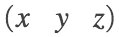div_F = divergence(F, [x y z])
div_F = 3

#### 10. [1109/22]

syms x y z f(y,z) g(x,z) h(x,y)
F(x,y,z) = [f(y,z) g(x,z) h(x,y)]
F(x, y, z) =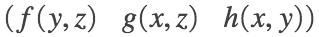div_F = divergence(F, [x y z])
div_F(x, y, z) = 0
% Since div(F) = 0, F is said to be incompressible (page 1107 of textbook).